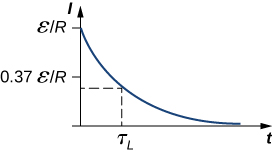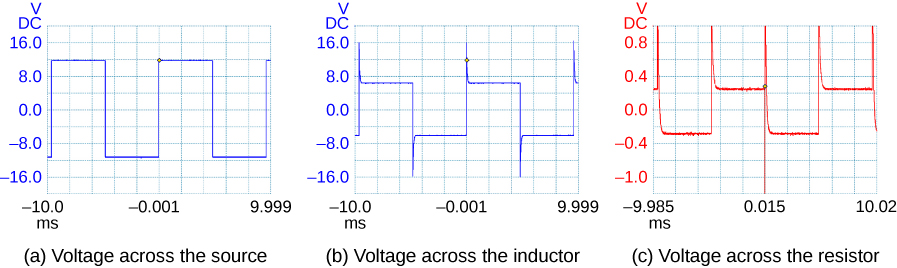# 14.4 Rl circuits  (Page 2/4)

 Page 2 / 4

The time constant ${\tau }_{L}$ also tells us how quickly the induced voltage decays. At $t={\tau }_{L},$ the magnitude of the induced voltage is

$|{V}_{L}\left({\tau }_{L}\right)|=\epsilon {e}^{-1}=0.37\epsilon =0.37V\left(0\right).$

The voltage across the inductor therefore drops to about $37\text{%}$ of its initial value after one time constant. The shorter the time constant ${\tau }_{L},$ the more rapidly the voltage decreases.

After enough time has elapsed so that the current has essentially reached its final value, the positions of the switches in [link] (a) are reversed, giving us the circuit in part (c). At $t=0,$ the current in the circuit is $I\left(0\right)=\epsilon \text{/}R.$ With Kirchhoff’s loop rule, we obtain

$IR+L\frac{dI}{dt}=0.$

The solution to this equation is similar to the solution of the equation for a discharging capacitor, with similar substitutions. The current at time t is then

$I\left(t\right)=\frac{\epsilon }{R}{e}^{\text{−}t\text{/}{\tau }_{L}}.$

The current starts at $I\left(0\right)=\epsilon \text{/}R$ and decreases with time as the energy stored in the inductor is depleted ( [link] ).

The time dependence of the voltage across the inductor can be determined from ${V}_{L}=\text{−}L\left(dI\text{/}dt\right)\text{:}$

${V}_{L}\left(t\right)=\epsilon {e}^{\text{−}t\text{/}\tau L}.$

This voltage is initially ${V}_{L}\left(0\right)=\epsilon$ , and it decays to zero like the current. The energy stored in the magnetic field of the inductor, $L{I}^{2}\text{/}2,$ also decreases exponentially with time, as it is dissipated by Joule heating in the resistance of the circuit.Time variation of electric current in the RL circuit of [link] (c). The induced voltage across the coil also decays exponentially.

## An RL Circuit with a source of emf

In the circuit of [link] (a), let $\epsilon =2.0V,R=4.0\phantom{\rule{0.2em}{0ex}}\text{Ω},\phantom{\rule{0.2em}{0ex}}\text{and}\phantom{\rule{0.2em}{0ex}}L=4.0\phantom{\rule{0.2em}{0ex}}\text{H}\text{.}\phantom{\rule{0.2em}{0ex}}$ With ${\text{S}}_{1}$ closed and ${\text{S}}_{2}$ open ( [link] (b)), (a) what is the time constant of the circuit? (b) What are the current in the circuit and the magnitude of the induced emf across the inductor at $t=0,\phantom{\rule{0.2em}{0ex}}\text{at}\phantom{\rule{0.2em}{0ex}}t=2.0{\tau }_{L}$ , and as $t\to \infty$ ?

## Strategy

The time constant for an inductor and resistor in a series circuit is calculated using [link] . The current through and voltage across the inductor are calculated by the scenarios detailed from [link] and [link] .

## Solution

1. The inductive time constant is
${\tau }_{L}=\frac{L}{R}=\frac{4.0\phantom{\rule{0.2em}{0ex}}\text{H}}{4.0\phantom{\rule{0.2em}{0ex}}\text{Ω}}=1.0\phantom{\rule{0.2em}{0ex}}\text{s}.$
2. The current in the circuit of [link] (b) increases according to [link] :
$I\left(t\right)=\frac{\text{ε}}{R}\left(1-{e}^{\text{−}t\text{/}{\tau }_{L}}\right).$

At $t=0,$
$\left(1-{e}^{\text{−}t\text{/}{\tau }_{L}}\right)=\left(1-1\right)=0;\phantom{\rule{0.2em}{0ex}}\text{so}\phantom{\rule{0.2em}{0ex}}I\left(0\right)=0.$

At $t=2.0{\tau }_{L}$ and $t\to \infty ,$ we have, respectively,
$I\left(2.0{\tau }_{L}\right)=\frac{\text{ε}}{R}\left(1-{e}^{-2.0}\right)=\left(0.50\phantom{\rule{0.2em}{0ex}}\text{A}\right)\left(0.86\right)=0.43\phantom{\rule{0.2em}{0ex}}\text{A},$

and
$I\left(\infty \right)=\frac{\text{ε}}{R}=0.50\phantom{\rule{0.2em}{0ex}}\text{A}.$

From [link] , the magnitude of the induced emf decays as
$|{V}_{L}\left(t\right)|=\text{ε}{e}^{\text{−}t\text{/}{\tau }_{L}}.$

$\text{At}\phantom{\rule{0.2em}{0ex}}t=0,t=2.0{\tau }_{L},\phantom{\rule{0.2em}{0ex}}\text{and as}\phantom{\rule{0.2em}{0ex}}t\to \infty ,$ we obtain
$\begin{array}{ccc}\hfill |{V}_{L}\left(0\right)|& =\hfill & \epsilon =2.0\phantom{\rule{0.2em}{0ex}}\text{V},\hfill \\ \hfill |{V}_{L}\left(2.0{\tau }_{L}\right)|& =\hfill & \left(2.0\phantom{\rule{0.2em}{0ex}}\text{V}\right)\phantom{\rule{0.2em}{0ex}}{e}^{-2.0}=0.27\phantom{\rule{0.2em}{0ex}}\text{V}\hfill \\ & \text{and}\hfill & \\ \hfill |{V}_{L}\left(\infty \right)|& =\hfill & 0.\hfill \end{array}$

## Significance

If the time of the measurement were much larger than the time constant, we would not see the decay or growth of the voltage across the inductor or resistor. The circuit would quickly reach the asymptotic values for both of these. See [link] .A generator in an RL circuit produces a square-pulse output in which the voltage oscillates between zero and some set value. These oscilloscope traces show (a) the voltage across the source; (b) the voltage across the inductor; (c) the voltage across the resistor.

## An RL Circuit without a source of emf

After the current in the RL circuit of [link] has reached its final value, the positions of the switches are reversed so that the circuit becomes the one shown in [link] (c). (a) How long does it take the current to drop to half its initial value? (b) How long does it take before the energy stored in the inductor is reduced to $1.0\text{%}$ of its maximum value?

## Strategy

The current in the inductor will now decrease as the resistor dissipates this energy. Therefore, the current falls as an exponential decay. We can also use that same relationship as a substitution for the energy in an inductor formula to find how the energy decreases at different time intervals.

## Solution

1. With the switches reversed, the current decreases according to
$I\left(t\right)=\frac{\epsilon }{R}{e}^{\text{−}t\text{/}{\tau }_{L}}=I\left(0\right){e}^{\text{−}t\text{/}{\tau }_{L}}.$

At a time t when the current is one-half its initial value, we have
$I\left(t\right)=0.50I\left(0\right)\phantom{\rule{0.2em}{0ex}}\text{so}\phantom{\rule{0.2em}{0ex}}{e}^{\text{−}t\text{/}{\tau }_{L}}=0.50,$

and
$t=\text{−}\left[\text{ln}\left(0.50\right)\right]{\tau }_{L}=0.69\left(1.0\phantom{\rule{0.2em}{0ex}}\text{s}\right)=0.69\phantom{\rule{0.2em}{0ex}}\text{s},$

where we have used the inductive time constant found in [link] .
2. The energy stored in the inductor is given by
${U}_{L}\left(t\right)=\frac{1}{2}L{\left[I\left(t\right)\right]}^{2}=\frac{1}{2}L{\left(\frac{\epsilon }{R}{e}^{\text{−}t\text{/}{\tau }_{L}}\right)}^{2}=\frac{L{\epsilon }^{2}}{2{R}^{2}}{e}^{-2t\text{/}{\tau }_{L}}.$

If the energy drops to $1.0\text{%}$ of its initial value at a time t , we have
${U}_{L}\left(t\right)=\left(0.010\right){U}_{L}\left(0\right)\phantom{\rule{0.2em}{0ex}}\text{or}\phantom{\rule{0.2em}{0ex}}\frac{L{\epsilon }^{2}}{2{R}^{2}}{e}^{-2t\text{/}{\tau }_{L}}=\left(0.010\right)\frac{L{\epsilon }^{2}}{2{R}^{2}}.$

Upon canceling terms and taking the natural logarithm of both sides, we obtain
$-\frac{2t}{{\tau }_{L}}=\text{ln}\left(0.010\right),$

so
$t=-\frac{1}{2}{\tau }_{L}\text{ln}\left(0.010\right).$

Since ${\tau }_{L}=1.0\phantom{\rule{0.2em}{0ex}}\text{s}$ , the time it takes for the energy stored in the inductor to decrease to $1.0\text{%}$ of its initial value is
$t=-\frac{1}{2}\left(1.0\phantom{\rule{0.2em}{0ex}}\text{s}\right)\text{ln}\left(0.010\right)=2.3\phantom{\rule{0.2em}{0ex}}\text{s}.$

## Significance

This calculation only works if the circuit is at maximum current in situation (b) prior to this new situation. Otherwise, we start with a lower initial current, which will decay by the same relationship.

#### Questions & Answers

how does beryllium decay occur
Photon?
Umar
state the first law of thermodynamics
Its state that "energy can neither be created nor destroyed but can be transformed from one form to another. "
Ayodamola
what about the other laws can anyone here help with it please
Sandy
The second law of thermodynamics states that the entropy of any isolated system always increases. The third law of thermodynamics states that the entropy of a system approaches a constant value as the temperature approaches absolute zero.
sahil
The first law is very simple to understand by its equation. The law states that "total energy in thermodynamic sytem is always constant" i.e d¶=du+dw where d¶=total heat du=internal energy dw=workdone... PLEASE REFER TO THE BOOKS FOR MORE UNDERSTANDING OF THE CONCEPT.
Elia
what is distance.?
what is physics?
Ali
Physics is a scientific phenomenon that deals with matter and its properties
Ayodamola
physics is the study of nature and science
John
Chater1to7
min
Physics is branch of science which deals with the study of matters in relation with energy.
Elia
What is differential form of Gauss's law?
help me out on this question the permittivity of diamond is 1.46*10^-10.( a)what is the dielectric of diamond (b) what its susceptibility
a body is projected vertically upward of 30kmp/h how long will it take to reach a point 0.5km bellow e point of projection
i have to say. who cares. lol. why know that t all
Jeff
is this just a chat app about the openstax book?
kya ye b.sc ka hai agar haa to konsa part
what is charge quantization
it means that the total charge of a body will always be the integral multiples of basic unit charge ( e ) q = ne n : no of electrons or protons e : basic unit charge 1e = 1.602×10^-19
Riya
is the time quantized ? how ?
Mehmet
What do you meanby the statement,"Is the time quantized"
Mayowa
Can you give an explanation.
Mayowa
there are some comment on the time -quantized..
Mehmet
time is integer of the planck time, discrete..
Mehmet
planck time is travel in planck lenght of light..
Mehmet
it's says that charges does not occur in continuous form rather they are integral multiple of the elementary charge of an electron.
Tamoghna
it is just like bohr's theory. Which was angular momentum of electron is intral multiple of h/2π
determine absolute zero
The properties of a system during a reversible constant pressure non-flow process at P= 1.6bar, changes from constant volume of 0.3m³/kg at 20°C to a volume of 0.55m³/kg at 260°C. its constant pressure process is 3.205KJ/kg°C Determine: 1. Heat added, Work done, Change in Internal Energy and Change in Enthalpy
U can easily calculate work done by 2.303log(v2/v1)
Abhishek
Amount of heat added through q=ncv^delta t
Abhishek
Change in internal energy through q=Q-w
Abhishek
please how do dey get 5/9 in the conversion of Celsius and Fahrenheit
what is copper loss
this is the energy dissipated(usually in the form of heat energy) in conductors such as wires and coils due to the flow of current against the resistance of the material used in winding the coil.
Henry
it is the work done in moving a charge to a point from infinity against electric field
what is the weight of the earth in space
As w=mg where m is mass and g is gravitational force... Now if we consider the earth is in gravitational pull of sun we have to use the value of "g" of sun, so we can find the weight of eaeth in sun with reference to sun...
Prince
g is not gravitacional forcé, is acceleration of gravity of earth and is assumed constante. the "sun g" can not be constant and you should use Newton gravity forcé. by the way its not the "weight" the physical quantity that matters, is the mass
Jorge
Yeah got it... Earth and moon have specific value of g... But in case of sun ☀ it is just a huge sphere of gas...
Prince
Thats why it can't have a constant value of g ....
Prince
not true. you must know Newton gravity Law . even a cloud of gas it has mass thats al matters. and the distsnce from the center of mass of the cloud and the center of the mass of the earth
JorgeByBy